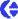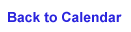### 19 March, 2002

Climate

Sunny, High 32, Low ñ7

The Science Behind Thermal Conductivity (keff),

continued

Snow density (p) is a measurement we use to calculate

thermal conductivity, which is one of two functions of

conductive heat flow. The other function is

Expressed in W/m/K (Watts per meter per Kelvin),

thermal conductivity is the ability to insulate and

conduct through an area. There are two equations used

depending on the mean snow density.

If the mean snow density is higher than .156

g/cm3
, then we use this equation.

(0.138 ñ 1.01p + 3.233p2)

If the mean snow density is equal or lower than

.156 g/cm3
, then this equation is used.

(.023 + .234p)

Letís Practice

Recall mean snow density you calculated on March 10th,

(.1649073 g/cm3). This value is higher than .156

g/cm3, therefore we use the first equation.

A. keff = (0.138 ñ 1.01p + 3.233p2)

B. keff = (0.138 ñ 1.01 (.1649073) +

3.233(.1649073)2)

C. keff = (0.138 - 0.1665563 + 0.0879195)

D. keff = 0.0593632 W/m/K

If the mean snow density is .1388112 g/cm3, what would

be the thermal conductivity? See tomorrowís journal to

On our way to Poker Flat along Steese Highway, the

view of Fairbanks becomes veiled with black and white

spruce trees as well as aspen, birch, and poplar

trees. Due to permafrost, tree roots extend

horizontally immediately below the surface. The result

of shallow roots, trees are often found lopsided.

__________________________________________________

Do You Yahoo!?

Yahoo! Movies - coverage of the 74th Academy AwardsÆ http://movies.yahoo.com/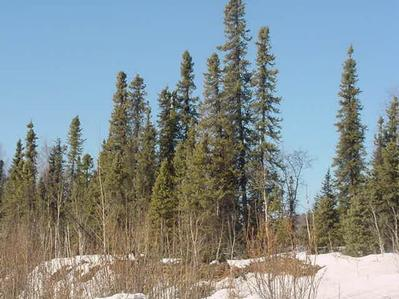Combination of black and white spruce trees.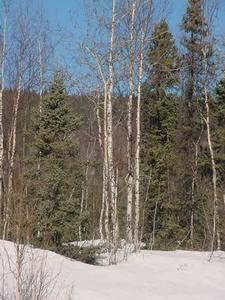Trees near Jalpertia pond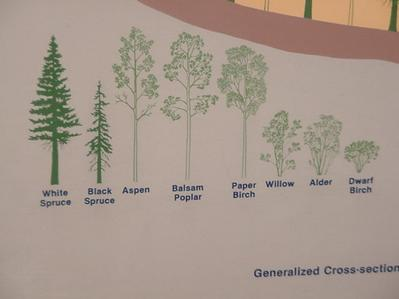Image from the UAF museumContact the TEA in the field at .
If you cannot connect through your browser, copy the TEA's e-mail address in the "To:" line of your favorite e-mail package.